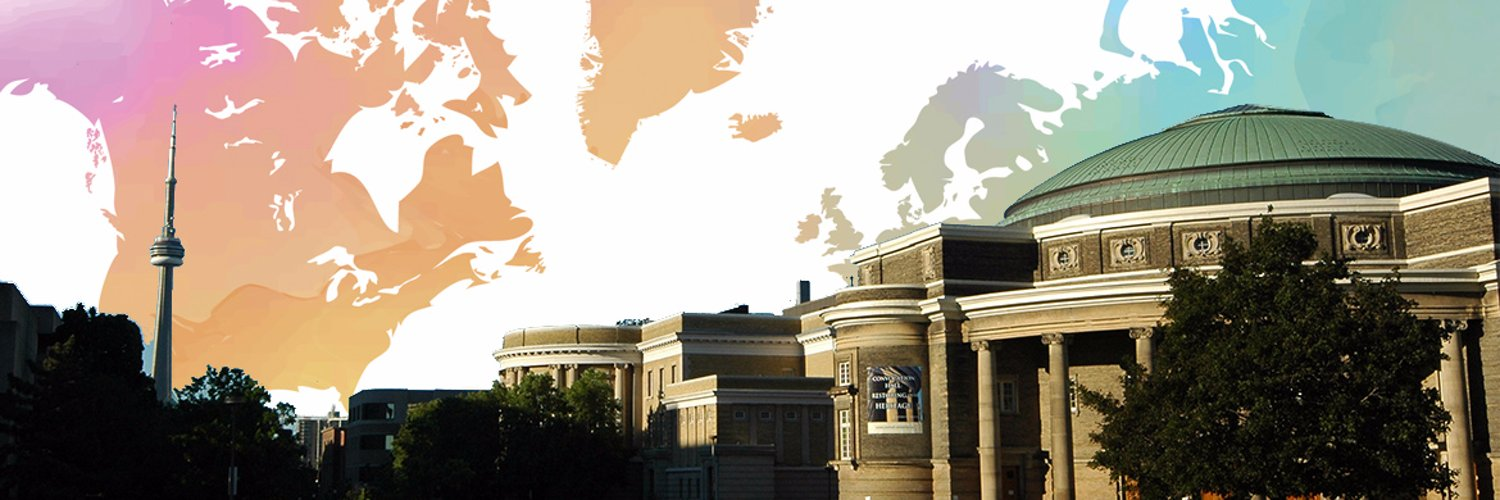Robert McCannProfessor | University of Toronto, Department of Mathematics

Professor McCann's research focuses on optimal transportation and its applications within and outside mathematics.

Biography

McCann grew up in Windsor Ontario, and studied engineering and physics at Queen's University before completing his doctorate in mathematics at Princeton in 1994. In the subsequent years, McCann has emerged as a leading figure in the development of the theory of optimal transportation. His work balances very pure contributions to deep mathematics with the discovery of new applications to image processing, atmospheric circulation patterns, and to optimizing economic decisions.

Before accepting a position at the University of Toronto, McCann was Tamarkin Assistant Professor of Mathematics at Brown University in Providence, Rhode Island.

Industry Applications (2)

Education/Learning Research

Research Interests (6)

Mathematical Physics Mathematical Economics Convex Analysis Geometry Optimization Partial Differential Equations

Accomplishments (2)

Coxeter-James Prize of the Canadian Mathematical Society (professional)

The Coxeter-James Prize was inaugurated to recognize young mathematicians who have made outstanding contributions to mathematical research. The first award was presented in 1978.

Monroe H. Martin Prize in Applied Mathematics (professional)

The prize will be awarded for an outstanding paper in applied mathematics (including numerical analysis) by a young research worker.

Affiliations (3)

• Fellow of the Royal Society of Canada (since 2014)
• Fellow of the Fields Institute (since 2015)
• Fellow of the American Mathematical Society (since 2012)

Articles (5)

Academic wages and pyramid schemes: a mathematical model Journal of Functional Analysis

2015

This paper analyzes a steady state matching model interrelating the education and labor sectors. In this model, a heterogeneous population of students match with teachers to enhance their cognitive skills. As adults, they then choose to become workers, managers, or teachers, who match in the labor or educational market to earn wages by producing output. We study the competitive equilibrium which results from the steady state requirement that the educational process replicate the same endogenous distribution of cognitive skills among ...

Multi-to one-dimensional transportation To appear in Communications on Pure and Applied Mathematics

2015

Fix probability densities f and g on open sets X⊂Rm and Y⊂Rn with m≥n≥1. Consider transporting f onto g so as to minimize the cost −s(x,y). We give a non-degeneracy condition (a) on s∈C1,1 which ensures the set of x paired with [g-a.e.] y∈Y lie in a codimension n submanifold of X. Specializing to the case m>n=1, we discover a nestedness criteria relating s to (f,g) which allows us to construct a unique optimal solution in the form of a map F:X⟶Y⎯⎯⎯⎯. When s∈C2∩W3,1 and logf and logg are bounded, the Kantorovich dual potentials (u,v) satisfy v∈C1,1loc(Y), and the normal velocity V of F−1(y) with respect to changes in y is given by V(x)=v"(f(x))−syy(x,f(x)). Positivity (b) of V locally implies a Lipschitz bound on f; moreover, v∈C2 if F−1(y) intersects ∂X∈C1 transversally (c)...

Dual potentials for capacity constrained optimal transport Calculus of Variations and Partial Differential Equations

2015

Optimal transportation with capacity constraints, a variant of the well-known optimal transportation problem, is concerned with transporting one probability density f ∈ L^ 1 (R^ m) f∈ L 1 (R m) onto another one g ∈ L^ 1 (R^ n) g∈ L 1 (R n) so as to optimize a cost function c ∈ L^ 1 (R^ m+ n) c∈ L 1 (R m+ n) while respecting the capacity constraints 0 ≤ h ≤ ̄ h ∈ L^ ∞ (R^ m+ n) 0≤ h≤ h¯∈ L∞(R m+ n). A linear programming duality for this problem was first proposed by Levin. In this note, we prove under mild assumptions on the ...

The intrinsic dynamics of optimal transport To appear in Journal de l'Ecole Polytechnique - Mathematiques

2015

The question of which costs admit unique optimizers in the Monge-Kantorovich problem of optimal transportation between arbitrary probability densities is investigated. For smooth costs and densities on compact manifolds, the only known examples for which the optimal solution is always unique require at least one of the two underlying spaces to be homeomorphic to a sphere. We introduce a (multivalued) dynamics which the transportation cost induces between the target and source space, for which the presence or absence of a ...

Higher-order time asymptotics of fast diffusion in Euclidean space: a dynamical systems approach Memoirs of the American Mathematical Society

2015

This paper quantifies the speed of convergence and higher-order asymptotics of fast diffusion dynamics on RnRn to the Barenblatt (self similar) solution. Degeneracies in the parabolicity of this equation are cured by re-expressing the dynamics on a manifold with a cylindrical end, called the cigar. The nonlinear evolution becomes differentiable in Hölder spaces on the cigar. The linearization of the dynamics is given by the Laplace-Beltrami operator plus a transport term (which can be suppressed by introducing appropriate weights into the function space norm), plus a finite-depth potential well with a universal profile...